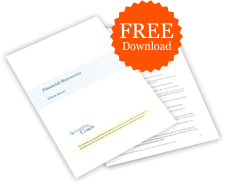# What is the double declining balance method of depreciation?

## Definition of Double Declining Balance Method of Depreciation

The double declining balance method of depreciation, also known as the 200% declining balance method of depreciation, is a form of accelerated depreciation. This means that compared to the straight-line method, the depreciation expense will be faster in the early years of the asset's life but slower in the later years. However, the total amount of depreciation expense during the life of the assets will be the same.

The "double" means 200% of the straight line rate of depreciation, while the "declining balance" refers to the asset's book value or carrying value at the beginning of the accounting period. Since book value is an asset's cost minus its accumulated depreciation, the asset's book value will be decreasing when the contra asset account Accumulated Depreciation is credited with the depreciation expense of the accounting period.

## Example of Double Declining Balance Depreciation

Let's assume that a retailer purchases fixtures on January 1 at a cost of \$100,000. It is expected that the fixtures will have no salvage value at the end of their useful life of 10 years. Under the straight-line method, the 10-year life means the asset's annual depreciation will be 10% of the asset's cost. Under the double declining balance method the 10% straight line rate is doubled to 20%. However, the 20% is multiplied times the fixture's book value at the beginning of the year instead of the fixture's original cost.

At the beginning of the first year, the fixture's book value is \$100,000 since the fixtures have not yet had any depreciation. Therefore, under the double declining balance method the \$100,000 of book value will be multiplied by 20% and will result in \$20,000 of depreciation for Year 1. The journal entry will be a debit of \$20,000 to Depreciation Expense and a credit of \$20,000 to Accumulated Depreciation.

At the beginning of the second year, the fixture's book value will be \$80,000, which is the cost of \$100,000 minus the accumulated depreciation of \$20,000. When the \$80,000 is multiplied by 20% the result is \$16,000 of depreciation for Year 2.

At the beginning of Year 3, the asset's book value will be \$64,000. This is the fixture's cost of \$100,000 minus its accumulated depreciation of \$36,000 (\$20,000 + \$16,000). The book value of \$64,000 multiplied by 20% is \$12,800 of depreciation expense for Year 3.

At the beginning of Year 4, the asset's book value will be \$51,200. Therefore, the book value of \$51,200 multiplied by 20% will result in \$10,240 of depreciation expense for Year 4.

At the beginning of Year 5, the asset's book value will be \$40,960. This is the amount to be depreciated over the remaining 6 years. In year 5, companies often switch to straight-line depreciation and debit Depreciation Expense and credit Accumulated Depreciation for \$6,827 (\$40,960/6 years) in each of the six remaining years.### Free Financial Statements Cheat Sheet

543,840
Subscribers
You are already subscribed. This offer is not available to existing subscribers.
Error: You have unsubscribed from this list.